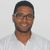# App Development

Announcements
cancel
Showing results for
Did you mean:Contributor III

## Splitting and adding String to Integer(Date)

Hello,

I have a field : (Years + Quarter)I have 2 questions:

1)how can I add a string in the middle of an integer

Example :(2018 3) to (2018 Q3).

2) How can i split the field to 2 parts

Example: (2018 3) to Year:(2018)    Quarter:(3)

Labels (4)

• ### String

2 Solutions

Accepted SolutionsMVP

for question 1 :

``left(Field,4)&' Q'&right(Field,1)``

for question2:

`` 'Year:('&left(Field,4)&') Quarter:('&right(Field,1)&')'``

for example :

``````Data:

load *, left(Field,4)&' Q'&right(Field,1) as Question1, 'Year:('&left(Field,4)&') Quarter:('&right(Field,1)&')' as Question2 inline [
Field
2018 1
2018 2
2018 3
2018 4
];``````

ouput:Regards,
Taoufiq ZARRA

"Please LIKE posts and "Accept as Solution" if the provided solution is helpful "

(you can mark up to 3 "solutions") 😉MVP

@Alarkis  like ?

`` 'Year:('&left(Field,4)&')' ``

and for quarter

``'Quarter:('&right(Field,1)&')'``

example :

``````Data:

load *, left(Field,4)&' Q'&right(Field,1) as Question1, 'Year:('&left(Field,4)&')' as Year, 'Quarter:('&right(Field,1)&')' as Quarter inline [
Field
2018 1
2018 2
2018 3
2018 4
];``````

output:Regards,
Taoufiq ZARRA

"Please LIKE posts and "Accept as Solution" if the provided solution is helpful "

(you can mark up to 3 "solutions") 😉
3 RepliesMVP

for question 1 :

``left(Field,4)&' Q'&right(Field,1)``

for question2:

`` 'Year:('&left(Field,4)&') Quarter:('&right(Field,1)&')'``

for example :

``````Data:

load *, left(Field,4)&' Q'&right(Field,1) as Question1, 'Year:('&left(Field,4)&') Quarter:('&right(Field,1)&')' as Question2 inline [
Field
2018 1
2018 2
2018 3
2018 4
];``````

ouput:Regards,
Taoufiq ZARRA

"Please LIKE posts and "Accept as Solution" if the provided solution is helpful "

(you can mark up to 3 "solutions") 😉Contributor III
Author

For question 2 i mean Year is separate field and Quarter is separate fieldMVP

@Alarkis  like ?

`` 'Year:('&left(Field,4)&')' ``

and for quarter

``'Quarter:('&right(Field,1)&')'``

example :

``````Data:

load *, left(Field,4)&' Q'&right(Field,1) as Question1, 'Year:('&left(Field,4)&')' as Year, 'Quarter:('&right(Field,1)&')' as Quarter inline [
Field
2018 1
2018 2
2018 3
2018 4
];``````

output:Regards,
Taoufiq ZARRA

"Please LIKE posts and "Accept as Solution" if the provided solution is helpful "

(you can mark up to 3 "solutions") 😉